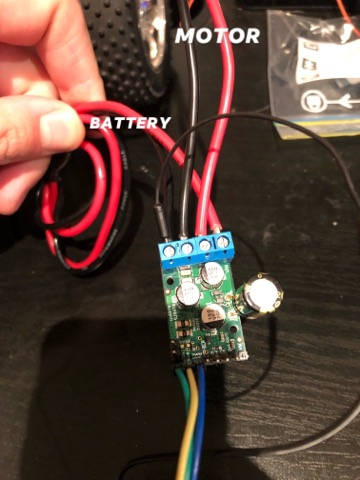# 18V25 With Raspberry Pi

Hi there,

I’m new to the forum and to robotics in general so forgive me if this problem is an easy fix.

I am currently trying to power a brushed DC motor with the Pololu 18V25 high powered motor driver (not the simple motor driver). The battery powering the motor (and thus the board I assume) is approximately 12V. I have attached a 220uf, 50V capacitor over the +,- terminal of the driver as I thought this was the spot it referred to on another forum post. I have then attached the DIR, PWM and SLP pins to the Raspberry Pi GPIO pins and connected the ground from the pi to the ground of the board containing the battery (I tried connecting it to the boards ground pin but this didnt work).

When I run the code I dont seem to be getting any voltage through the board despite the battery being fully charged and thus the wheels dont spin.

I have attached some pictures and my code below for reference. Thank you``````import pandas as pd
import time
from time import sleep
import numpy as np
import RPi.GPIO as GPIO

#Defining all the Pins
PWM=24
DIR=23
SLP=25
Car_Max_Speed=50 #km/h

GPIO.setmode(GPIO.BCM)
GPIO.setup(PWM,GPIO.OUT)
GPIO.setup(DIR,GPIO.OUT)
GPIO.setup(SLP,GPIO.OUT)
GPIO.output(PWM,GPIO.LOW)
GPIO.output(DIR,GPIO.LOW)

voltage=GPIO.PWM(SLP,1000) #enable pin, 1000Hz
#startup=voltage.start(25)

##Asking the User to Select the Function
print ("Welcome to the ZoomyBoi. The device has two functions: a constant speed 'Training' function or a 'Race' simulation function.")

##RACE SIM MODE
if function=='Race':
print ("You have entered the race simulation mode.")
print ("Prior to running in this mode, please ensure your split times are saved as excel file (.xlsx) with a '_' before the title.")
print ("Split times should list distance first followed by time.")
#Importing the Split File
while True:
try:
filename=input("Enter the File Name here: ")
PATH='_'+filename+'.xlsx'
break
except FileNotFoundError:
print('No such file.  Check file name and path and try again.')

times=df.iloc[:,1] #extracting the split times
length=len(times) #array length
split_distance=df.iloc[1,0]-df.iloc[0,0]

omega=np.zeros(length)

#Calculating the necessary motor speed based on a percentage of the car's max speed.
for i in range(1, length, 1): #populates velocity array

lin_speed = round(3.6*(split_distance/times[i]),2) #linear speed in km/h
omega[i]=100*(lin_speed/Car_Max_Speed) #Finding Duty cycle as a percentage of max speed
print(omega[i])

if i == len(omega)-1:
print("Array Full...")
break

for j in range(0, length, 1):

print("Motor spinning up...")
print(j)
time.sleep(times[j])
GPIO.output(DIR, GPIO.HIGH)
GPIO.output(PWM, GPIO.HIGH)
voltage.ChangeDutyCycle(omega[i])

if j == len(omega)-1:
print("Trial Completed...")
GPIO.output(PWM, GPIO.LOW)
GPIO.output(DIR, GPIO.LOW)
break

##CONSTANT SPEED MODE
elif function=='Training':
print ("You have entered the training mode where the vehicle operates at a constant speed.")
dist=int(input("Please enter the distance you would like to travel in metres: "))
time=int(input("Please enter the time you wish to complete this run in seconds: "))
alpha=np.zeros(time)
arraylength=len(alpha)
linearspeed=((dist/time)*3.6) #linear speed in km/h
print(alpha)

import time
from time import sleep

if linearspeed >= Car_Max_Speed:
print("Sorry, Zoomyboi cant go that fast. Please run the program again and try a different speed.")

else:

for k in range(1, arraylength, 1): #populates velocity array

alpha[k]=100*(linearspeed/Car_Max_Speed) #Finding Duty cycle as a percentage of max speed
print(alpha[k])

if k == len(alpha)-1:
print("Array Full...")

for l in range(0, arraylength, 1):

print("Motor spinning up...")
print(l)
time.sleep(1)
GPIO.output(DIR, GPIO.HIGH)
GPIO.output(PWM, GPIO.HIGH)
voltage.ChangeDutyCycle(alpha[k])

if l == len(alpha)-1:
print("Trial Completed...")
GPIO.output(DIR,GPIO.LOW)
GPIO.output(PWM,GPIO.LOW)
break

else:
print ("Invalid input. Please run the program again.")

``````

I should mention that the code is one that I used from an old L298N driver board which I had to replace. It worked fine with that so I assumed it wouldnt have an issue with this board.

I managed to get it to work! just had a few issues in the code (i.e. the SLP pin wasnt needed)

Hello,

I’m glad you figured out your problem; thank you for following up to let us know you got it working!

-Dan